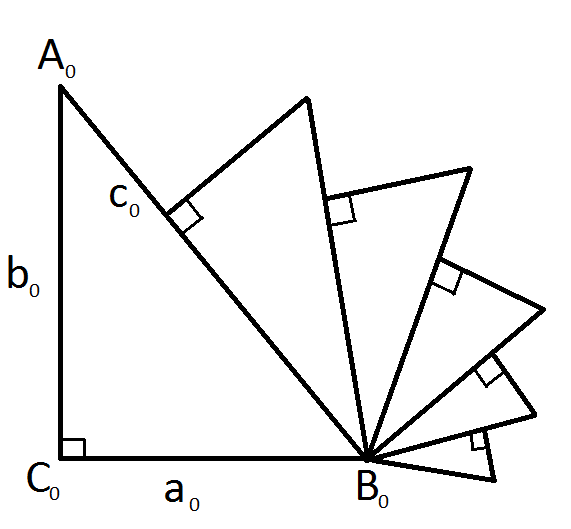# Nesting some triangles

Geometry Level 3Given that right $\Delta A_0B_0C_0$ has legs $a_0$ and $b_0$ such that $a_0=b_0=2$. Another right triangle $\Delta A_1B_0C_1$ is drawn from the hypotenuse of $\Delta A_0B_0C_0$ such that its non-adjacent perpendicular side is drawn $\frac{2}{3} c_0$ from $B_0$. Then, another right triangle $\Delta A_2B_0C_2$ is drawn from the hypotenuse of $\Delta A_1B_0C_1$ such that its non-adjacent perpendicular side is drawn $\frac{2}{3} c_1$ from $B_0$ and so on, as is depicted by the image above.

If all the triangles drawn are similar to one another, what is the sum of areas of all these triangles drawn up to infinity?

Clarification: Uppercase letters denote vertices; lowercase letters denote side lengths.

×